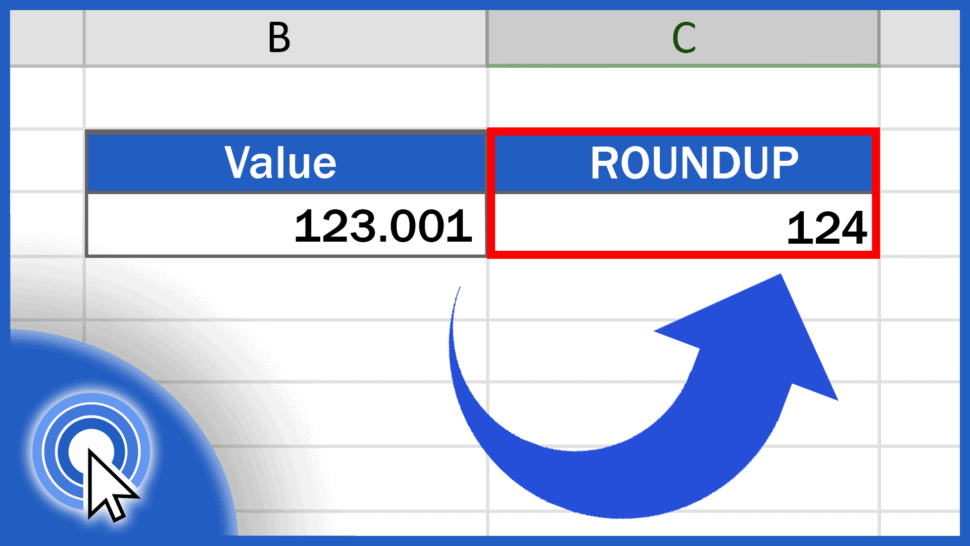# How to ROUNDUP in ExcelIn today’s tutorial, we’re going to talk about numbers, specifically how to round up in Excel. Thanks to the ROUNDUP function, Excel will always round values up, based on the given number of decimal places.

Together, we’ll have a look at how to use this function effectively. Let’s start now!

See the video tutorial and transcription below:

Contents

As we already know, Excel offers multiple functions when it comes to calculations of any kind. The same goes for rounding numbers.

We can round up, round down, or simply round numbers according to the general mathematical rule.

Each function will help you round numbers in a different way and each is going to be presented in a separate tutorial. You’ll find them here.

In this tutorial, we’re gonna have a closer look at the function ROUNDUP.

## How to ROUNDUP to one decimal place in Excel

Let’s say we want to round up the value in the column B to various numbers of decimal places or to make it a whole number.

Click into the cell C3, enter the equal sign and type in the function ‘ROUNDUP’. Then carry on with an opening bracket and here we’ll need to include two things.

The first one will be the cell which contains the number we want to round up, so click on B3, then enter a comma and you can type in the second value – the number which identifies how many decimal places there should be in the result.

So, if you want to round up to one decimal place, type ‘1’, close the parentheses, and hit ‘Enter’. In C3, Excel will show the result – the number from B3 rounded up to one decimal place.

## How to ROUNDUP to two decimal places in Excel

If you want to round up to two decimal places, simply use 2 in the function and the resulting number will be rounded up to two decimal places.

By using positive numbers like 1, 2, 3, and so on, you can round up to any number of decimal places.

But let’s move on, now.

## How to round the value up to make it a whole number

To round the value up to make it a whole number, enter a zero in the function, and Excel will do just what you want – it will round the original value up making it a whole number, like this.

And we’re not finished yet!

## How to round a number up to tens or hundreds

To round a number up to tens or hundreds, which means moving to the left from the decimal point,

you need to use negative numbers in the formula, so make sure you include the minus sign with the number in parentheses.

Here are a couple of examples.

To round the number up to the nearest ten, enter ‘-1’ into the formula and here comes the result!

If you want to round the number up to the nearest hundred, type in ‘-2’ and Excel will calculate it for you immediately.

And that’s how it works! You can use this function to round up any number just as you need.If you’d like to know more about similar functions, like the function Round or Round Down, you can learn how to use them effectively by watching the rest of the video tutorials on rounding numbers by EasyClick Academy. Check out the links in the description below!

If you found this tutorial helpful, give us a like and watch other video tutorials by EasyClick Academy. Learn how to use Excel in a quick and easy way!

Is this your first time on EasyClick? We’ll be more than happy to welcome you in our online community. Hit that Subscribe button and join the EasyClickers!

Thanks for watching and I’ll see you in the next tutorial!